# Three.js实现穿越云层动效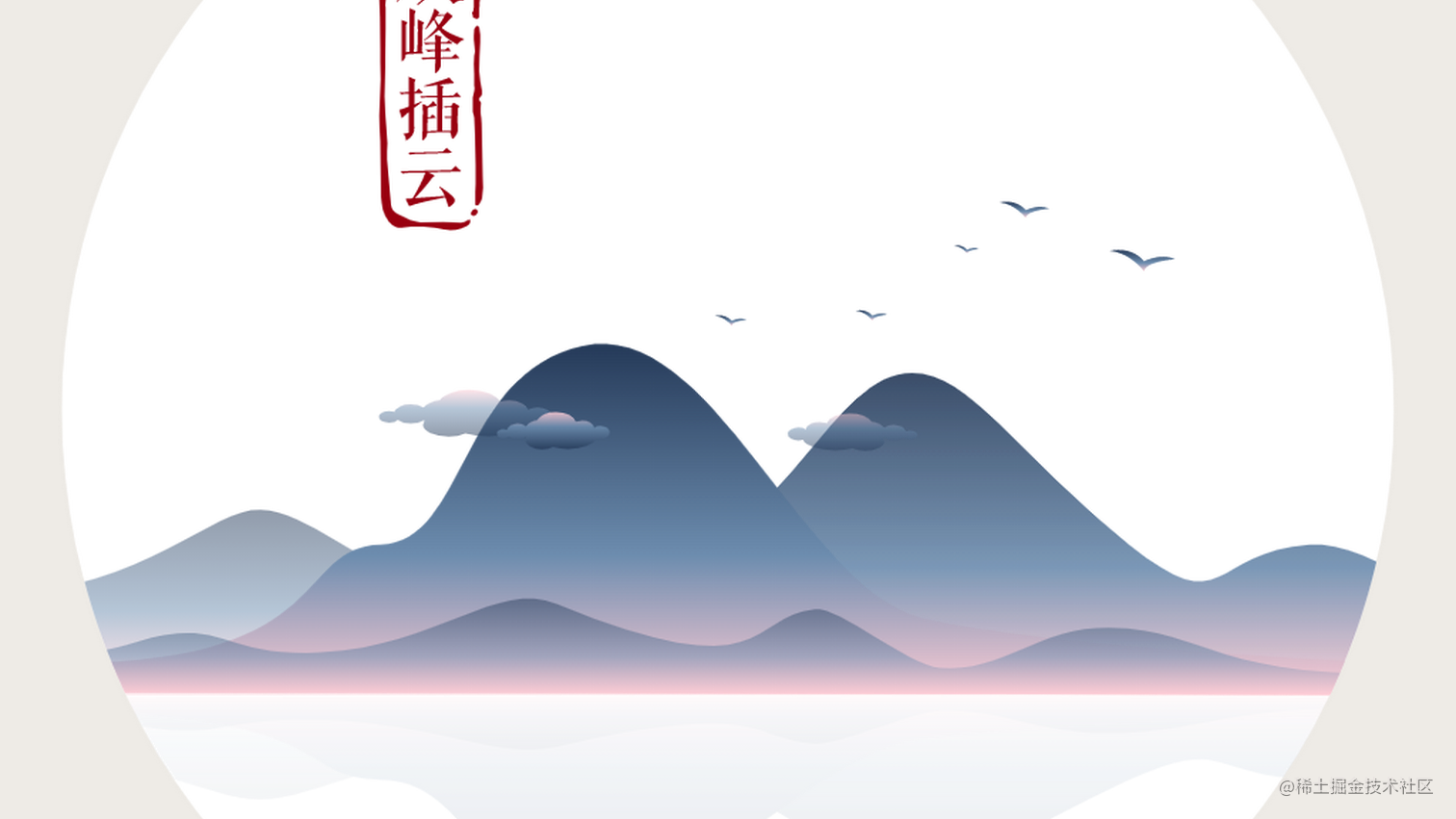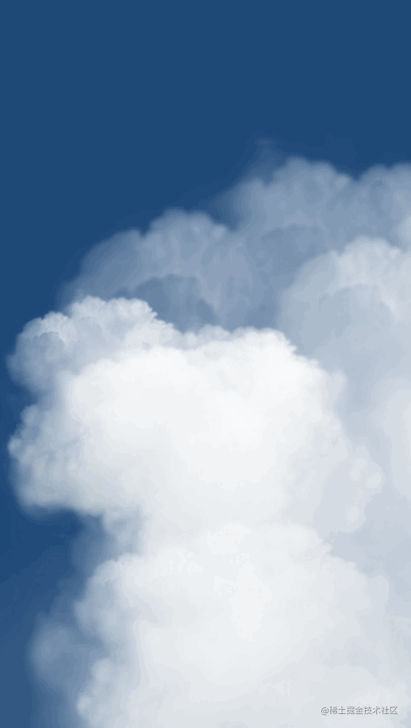1. 沿着 Z 轴均匀的放一堆 64*64 的平面图形，这些平面的 X 坐标和 Y 坐标是随机的（很像下图的桶装薯片）
2. 把上面的所有图形合并成一个大的图形
3. 把大的图形和贴片材质（云）生成网格，网格放进场景中
4. 动效就是将相机从远处沿着 Z 轴缓慢移动，就会有了穿越云层的效果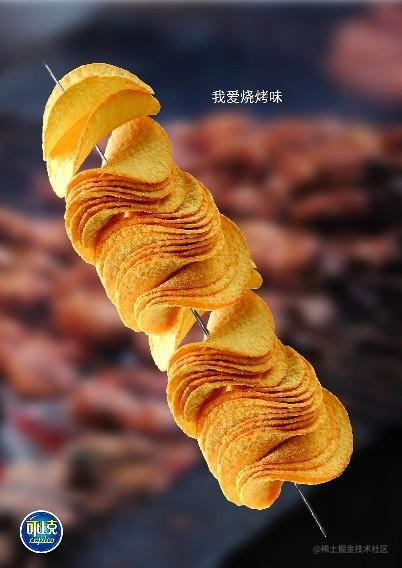## 场景

``````// 初始化场景
var scene = new THREE.Scene();

// 其他代码...
// 把物体添加进场景
// 渲染场景
renderer.render(scene, camera);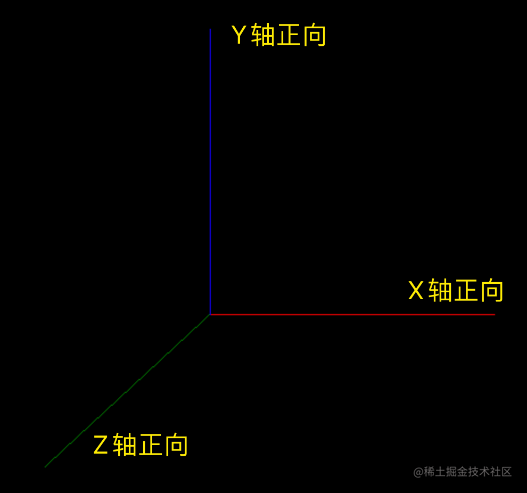``````const scene = new THREE.Scene();

var camera = new THREE.PerspectiveCamera(70, window.innerWidth / window.innerHeight, 1, 1000);
camera.position.set(0, 0, 100);

const renderer = new THREE.WebGLRenderer();
renderer.setSize(window.innerWidth, window.innerHeight);
document.body.appendChild(renderer.domElement);

// 线段1，红色的，从原点到X轴40
const points = [];
points.push(new THREE.Vector3(0, 0, 0));
points.push(new THREE.Vector3(40, 0, 0));
const geometry1 = new THREE.BufferGeometry().setFromPoints(points);
var material1 = new THREE.LineBasicMaterial({ color: 'red' });
var line1 = new THREE.Line(geometry1, material1);

// 线段2，蓝色的，从原点到Y轴40
points.length = 0;
points.push(new THREE.Vector3(0, 0, 0));
points.push(new THREE.Vector3(0, 40, 0));
const geometry2 = new THREE.BufferGeometry().setFromPoints(points);
var material2 = new THREE.LineBasicMaterial({ color: 'blue' });
var line2 = new THREE.Line(geometry2, material2);

// 线段3，绿色的，从原点到Z轴40
points.length = 0;
points.push(new THREE.Vector3(0, 0, 0));
points.push(new THREE.Vector3(0, 0, 40));
const geometry3 = new THREE.BufferGeometry().setFromPoints(points);
var material3 = new THREE.LineBasicMaterial({ color: 'green' });
var line3 = new THREE.Line(geometry3, material3);
// 做了个旋转，不然看不到Z轴上的线
line3.rotateX(Math.PI / 8);
line3.rotateY(-Math.PI / 8);

renderer.render(scene, camera);

## 相机

``````// 初始化相机
camera = new THREE.PerspectiveCamera(70, pageWidth / pageHeight, 1, 1000);

// 最后，场景和相机一起渲染出来，我们就能够看到场景中的物体了
renderer.render(scene, camera);

## 材质

``````// 贴图材质
// 这里的值是给着色器传递的
uniforms: {
map: {
type: 't',
value: texture
},
fogColor: {
type: 'c',
value: fog.color
},
fogNear: {
type: 'f',
value: fog.near
},
fogFar: {
type: 'f',
value: fog.far
}
},
transparent: true
});

## 图形和网格

`Three.js`默认提供了很多的几何体图形，也就是各种`Geometry`，他们的基类是`BufferGeometry`

``````// 把上面合并出来的形状和材质，生成一个网格
mesh = new THREE.Mesh(mergedGeometry, material);

## 渲染

``````function animate() {
requestAnimationFrame(animate);
renderer.render(scene, camera);
}

## 揭秘过程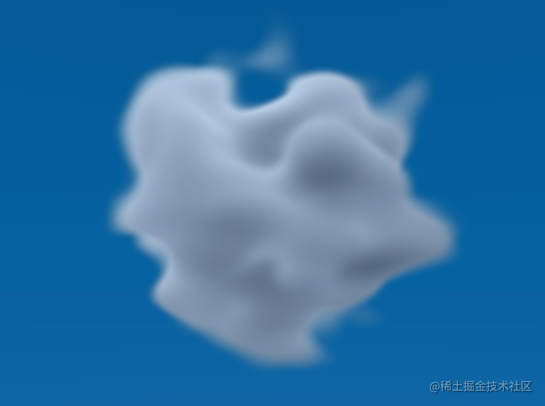### THREE.Geometry

``````// 初始化一个基础的图形
geometry = new THREE.Geometry();
// 初始化一个64*64的平面
var plane = new THREE.Mesh(new THREE.PlaneGeometry(64, 64));

for (var i = 0; i < 8000; i++) {
// 调整平面图案的位置和旋转角度等
plane.position.x = Math.random() * 1000 - 500;
plane.position.y = -Math.random() * Math.random() * 200 - 15;
plane.position.z = i;
plane.rotation.z = Math.random() * Math.PI;
plane.scale.x = plane.scale.y = Math.random() * Math.random() * 1.5 + 0.5;
// 平面合并到基础图形
THREE.GeometryUtils.merge(geometry, plane);
}

``````// 一个平面形状
const geometry = new THREE.PlaneGeometry(64, 64);
const geometries = [];

for (var i = 0; i < CloudCount; i++) {
const instanceGeometry = geometry.clone();

// 把这个克隆出来的云，通过随机参数，做一些位移，达到一堆云彩的效果，每次渲染出来的云堆都不一样
// X轴偏移后，通过调整相机位置达到平衡
// Y轴想把云彩放在场景的偏下位置，所以都是负值
// Z轴位移就是：当前第几个云*每个云所占的Z轴长度
instanceGeometry.translate(Math.random() * RandomPositionX, -Math.random() * RandomPositionY, i * perCloudZ);

geometries.push(instanceGeometry);
}

// 把这些形状合并
const mergedGeometry = BufferGeometryUtils.mergeBufferGeometries(geometries);

### GeometryUtils.merge

``````// 合并所有的平面图形到一个基础图形
THREE.GeometryUtils.merge(geometry, plane);

``````// 把这些形状合并
const mergedGeometry = BufferGeometryUtils.mergeBufferGeometries(geometries);

### 着色器

``````// 原来的
varying vec2 vUv;
void main()
{
vUv = uv;
gl_Position = projectionMatrix * modelViewMatrix * vec4( position, 1.0 );
}
</script>

uniform sampler2D map;
uniform vec3 fogColor;
uniform float fogNear;
uniform float fogFar;
varying vec2 vUv;
void main()
{
float depth = gl_FragCoord.z / gl_FragCoord.w;
float fogFactor = smoothstep( fogNear, fogFar, depth );
gl_FragColor = texture2D(map, vUv );
gl_FragColor.w *= pow( gl_FragCoord.z, 20.0 );
gl_FragColor = mix( gl_FragColor, vec4( fogColor, gl_FragColor.w ), fogFactor );
}
</script>

``````const vShader = `
varying vec2 vUv;
void main()
{
vUv = uv;
gl_Position = projectionMatrix * modelViewMatrix * vec4( position, 1.0 );
}
`;